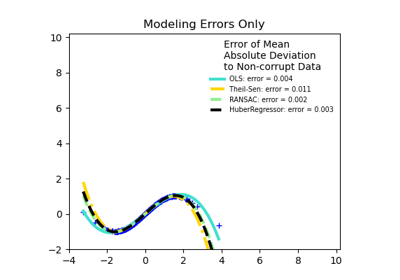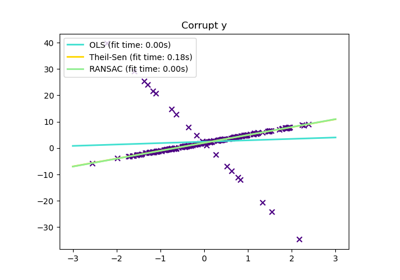# sklearn.linear_model.TheilSenRegressor¶

class sklearn.linear_model.TheilSenRegressor(*, fit_intercept=True, copy_X=True, max_subpopulation=10000.0, n_subsamples=None, max_iter=300, tol=0.001, random_state=None, n_jobs=None, verbose=False)[source]

Theil-Sen Estimator: robust multivariate regression model.

The algorithm calculates least square solutions on subsets with size n_subsamples of the samples in X. Any value of n_subsamples between the number of features and samples leads to an estimator with a compromise between robustness and efficiency. Since the number of least square solutions is “n_samples choose n_subsamples”, it can be extremely large and can therefore be limited with max_subpopulation. If this limit is reached, the subsets are chosen randomly. In a final step, the spatial median (or L1 median) is calculated of all least square solutions.

Read more in the User Guide.

Parameters:
fit_interceptbool, default=True

Whether to calculate the intercept for this model. If set to false, no intercept will be used in calculations.

copy_Xbool, default=True

If True, X will be copied; else, it may be overwritten.

max_subpopulationint, default=1e4

Instead of computing with a set of cardinality ‘n choose k’, where n is the number of samples and k is the number of subsamples (at least number of features), consider only a stochastic subpopulation of a given maximal size if ‘n choose k’ is larger than max_subpopulation. For other than small problem sizes this parameter will determine memory usage and runtime if n_subsamples is not changed. Note that the data type should be int but floats such as 1e4 can be accepted too.

n_subsamplesint, default=None

Number of samples to calculate the parameters. This is at least the number of features (plus 1 if fit_intercept=True) and the number of samples as a maximum. A lower number leads to a higher breakdown point and a low efficiency while a high number leads to a low breakdown point and a high efficiency. If None, take the minimum number of subsamples leading to maximal robustness. If n_subsamples is set to n_samples, Theil-Sen is identical to least squares.

max_iterint, default=300

Maximum number of iterations for the calculation of spatial median.

tolfloat, default=1e-3

Tolerance when calculating spatial median.

random_stateint, RandomState instance or None, default=None

A random number generator instance to define the state of the random permutations generator. Pass an int for reproducible output across multiple function calls. See Glossary.

n_jobsint, default=None

Number of CPUs to use during the cross validation. None means 1 unless in a joblib.parallel_backend context. -1 means using all processors. See Glossary for more details.

verbosebool, default=False

Verbose mode when fitting the model.

Attributes:
coef_ndarray of shape (n_features,)

Coefficients of the regression model (median of distribution).

intercept_float

Estimated intercept of regression model.

breakdown_float

Approximated breakdown point.

n_iter_int

Number of iterations needed for the spatial median.

n_subpopulation_int

Number of combinations taken into account from ‘n choose k’, where n is the number of samples and k is the number of subsamples.

n_features_in_int

Number of features seen during fit.

New in version 0.24.

feature_names_in_ndarray of shape (n_features_in_,)

Names of features seen during fit. Defined only when X has feature names that are all strings.

New in version 1.0.

HuberRegressor

Linear regression model that is robust to outliers.

RANSACRegressor

RANSAC (RANdom SAmple Consensus) algorithm.

SGDRegressor

Fitted by minimizing a regularized empirical loss with SGD.

References

Examples

>>> from sklearn.linear_model import TheilSenRegressor
>>> from sklearn.datasets import make_regression
>>> X, y = make_regression(
...     n_samples=200, n_features=2, noise=4.0, random_state=0)
>>> reg = TheilSenRegressor(random_state=0).fit(X, y)
>>> reg.score(X, y)
0.9884...
>>> reg.predict(X[:1,])
array([-31.5871...])


Methods

 fit(X, y) Fit linear model. get_params([deep]) Get parameters for this estimator. Predict using the linear model. score(X, y[, sample_weight]) Return the coefficient of determination of the prediction. set_params(**params) Set the parameters of this estimator.
fit(X, y)[source]

Fit linear model.

Parameters:
Xndarray of shape (n_samples, n_features)

Training data.

yndarray of shape (n_samples,)

Target values.

Returns:
selfreturns an instance of self.

Fitted TheilSenRegressor estimator.

get_params(deep=True)[source]

Get parameters for this estimator.

Parameters:
deepbool, default=True

If True, will return the parameters for this estimator and contained subobjects that are estimators.

Returns:
paramsdict

Parameter names mapped to their values.

predict(X)[source]

Predict using the linear model.

Parameters:
Xarray-like or sparse matrix, shape (n_samples, n_features)

Samples.

Returns:
Carray, shape (n_samples,)

Returns predicted values.

score(X, y, sample_weight=None)[source]

Return the coefficient of determination of the prediction.

The coefficient of determination $$R^2$$ is defined as $$(1 - \frac{u}{v})$$, where $$u$$ is the residual sum of squares ((y_true - y_pred)** 2).sum() and $$v$$ is the total sum of squares ((y_true - y_true.mean()) ** 2).sum(). The best possible score is 1.0 and it can be negative (because the model can be arbitrarily worse). A constant model that always predicts the expected value of y, disregarding the input features, would get a $$R^2$$ score of 0.0.

Parameters:
Xarray-like of shape (n_samples, n_features)

Test samples. For some estimators this may be a precomputed kernel matrix or a list of generic objects instead with shape (n_samples, n_samples_fitted), where n_samples_fitted is the number of samples used in the fitting for the estimator.

yarray-like of shape (n_samples,) or (n_samples, n_outputs)

True values for X.

sample_weightarray-like of shape (n_samples,), default=None

Sample weights.

Returns:
scorefloat

$$R^2$$ of self.predict(X) wrt. y.

Notes

The $$R^2$$ score used when calling score on a regressor uses multioutput='uniform_average' from version 0.23 to keep consistent with default value of r2_score. This influences the score method of all the multioutput regressors (except for MultiOutputRegressor).

set_params(**params)[source]

Set the parameters of this estimator.

The method works on simple estimators as well as on nested objects (such as Pipeline). The latter have parameters of the form <component>__<parameter> so that it’s possible to update each component of a nested object.

Parameters:
**paramsdict

Estimator parameters.

Returns:
selfestimator instance

Estimator instance.

## Examples using sklearn.linear_model.TheilSenRegressor¶Robust linear estimator fitting

Robust linear estimator fittingTheil-Sen Regression

Theil-Sen Regression